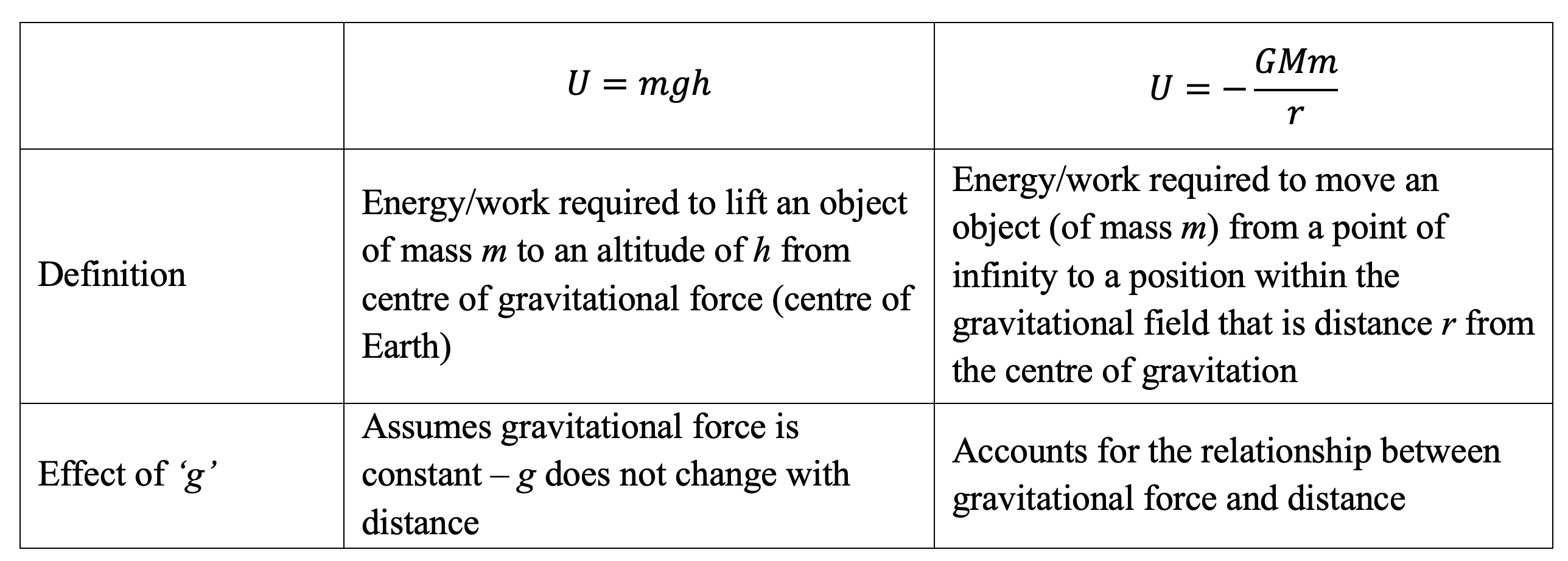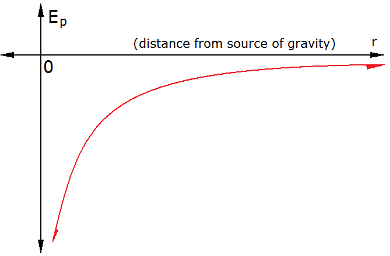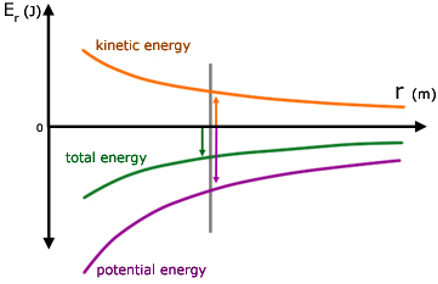# M5-S11: Gravitational Potential Energy, Energy Changes in Space and Escape Velocity

• ### Derive quantitatively and apply the concepts of gravitational force and gravitational potential energy in radial gravitational fields to a variety of situations, including but not limited to:

• the concept of escape velocity v_(esc)=sqrt((2GM)/r)
• total potential energy of a planet or satellite in its orbit U=-(GMm)/r
• total energy of a planet or satellite in its orbit U+K=-(GMm)/(2r)
• energy changes that occur when satellites move between orbits
• Kepler’s Laws of Planetary Motion

### Gravitational Potential Energy and Work Done in Space

• All objects experience gravitational force when they are within a gravitational field

All objects possess gravitational potential energy which is defined as the potential amount of work done by gravity for a certain displacement s

U=mgh

• However, as the gravitational force varies with distance of separation, a new and more accurate equation needs to be devised:
U=-(GMm)/rNote: the reason why we define the equation using an infinity point because it is consistent regardless of an object’s position in the gravitational field. At distance oo, Ep approaches 0, as we move the object from this point, the value of r decreases, and theoretically its potential energy should also decrease. Therefore, as a result of how we define the equation, the potential energy becomes more negative.• Gravitational potential energy is inversely proportional to orbital radius.
• Work done to move an object against gravity equals to change in potential energy of the object during the movement.
W = DeltaU = U_f - U_i

W = -(GMm)/(r_f)+(GMm)/(r_i)

Practice Question 4

Define the term Gravitational Potential Energy.

Practice Question 5

A satellite with a mass of 200 kg maintains its orbit at an altitude of 300 km above the Earth’s surface. The Earth has a radius of 6.371 x 106 m and a mass of 6.00 x 1024 kg.

(a) Determine the gravitational potential energy of the satellite at this altitude. (2 marks.

(b) Calculate the work done to move this satellite to an altitude of 4000 km above Earth’s surface. (2 marks)

Kinetic Energy

Besides possessing gravitational potential energy, all objects in motion in space possess kinetic energy given by the equation:

K=1/2mv^2

By substitution velocity as orbital velocity:

K=1/2m(sqrt((GM)/r))^2

K=(GMm)/(2r)

• The orbital velocity of an object (satellite or planet) decreases as the orbital radius increases. Thus, the kinetic energy of these objects also decreases.

Total Energy

• Total mechanical energy (T) of an object in orbit equals to sum of its kinetic energy (K) and gravitational potential energy (U)
T = K + U

T= (GMm)/(2r) – (GMm)/r

T = - (GMm)/(2r)

T = 1/2U• Therefore, total energy of an object in orbital motion is exactly half of its gravitational potential energy (see graph).
• If the propeller or thrust of a spacecraft or satellite is turned off, the total mechanical energy remains constant throughout its orbit. Of course, this is assuming frictional forces are negligible.

### Change in Energy Within and Between Orbits

• Total energy remains constant and the law of conservation of energy applies.
• Kepler’s first law states that an orbit around a planetary or stellar body is elliptical. This means the radius of orbit changes.
• As an orbiting object decreases in altitude or orbital radius r, its gravitational potential energy decreases and is transformed into kinetic energy. Thus, kinetic energy increases.

This is consistent with an increase in orbital velocity

• As an orbiting object increases in altitude or orbital radius r, its orbital velocity decreases and so does its kinetic energy. The reduction of kinetic energy means gravitational energy increases.

### Escape Velocity

Imagine an object travelling faraway enough to finally escape the effect of gravity. By Newton’s universal law of gravitation, this scenario can only occur at r = oo

• As a mass travels away from the centre of a gravitational field, its kinetic energy is converted to gravitational potential energy. Therefore, kinetic energy decreases while gravitational potential energy increases.
• At r = oo both gravitational potential and kinetic energy of the object both become zero. Therefore, the total energy also becomes zero.
• By using law of conservation of energy:

K+U=0

1/2mv^2=(GMm)/r

v^2=(2GM)/r

v_(esc)=sqrt((2GM)/r)

We can determine the minimal velocity required to allow an object to escape the gravitational field it is in.

• Do not confused this equation with orbital velocity – they are fundamentally very different concepts.
• Escape velocity is always greater than orbital velocity because more kinetic energy is needed to ‘overcome’ the gravitational force than to accompany it.

Practice Question 6

A satellite of mass 1000 kg has been launched from the surface of Earth and sometime later then potential energy of the satellite was determined to be – 3.97 J. Determine the distance of the satellite from Earth at this point and hence the escape velocity of the satellite (4 marks)

Practice Question 7

Compare the escape velocity of low-Earth and geostationary satellites of equal mass (2 marks)

Previous section: Kepler's Laws of Planetary Motion

Next section: Low Earth and Geostationary Orbit Satellites